Function Repository Resource:

# SubsetFromIndex

Get the subset with the given index and length

Contributed by: Ed Pegg Jr
 ResourceFunction["SubsetFromIndex"][index,len] returns a subset of length len with given index.

## Details and Options

Subsets are considered to be lists {a1,a2,,ak} such that the ai are non-negative integers and strictly increasing.
Subsets are sorted by their reverse; {1,2,3} comes before {0,1,4}.
For any subset, a unique index n can be defined via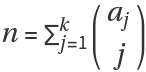.
For example: subset {2,4,6} has index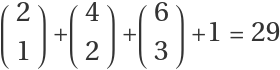.

## Examples

### Basic Examples (3)

Give the first ten 3-subsets:

 In:=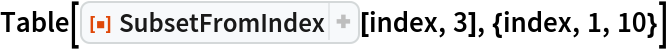Out=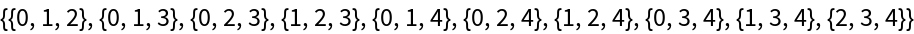The following code gives the same 3-subset sequence:

 In:=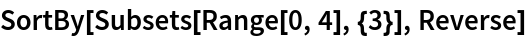Out=The function can return subsets of a large index:

 In:=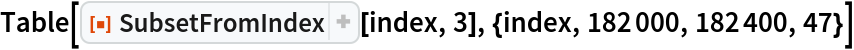Out=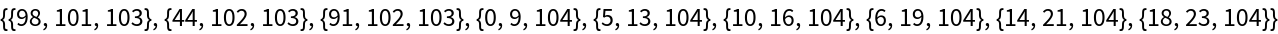The function can return the subset of a given index for various lengths:

 In:=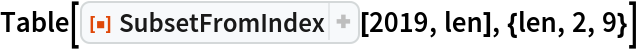Out=### Scope (3)

Some binomial representations of the number 320:

 In:=Out=Here are some 2-subsets with their indices to show their structure:

 In:=Out=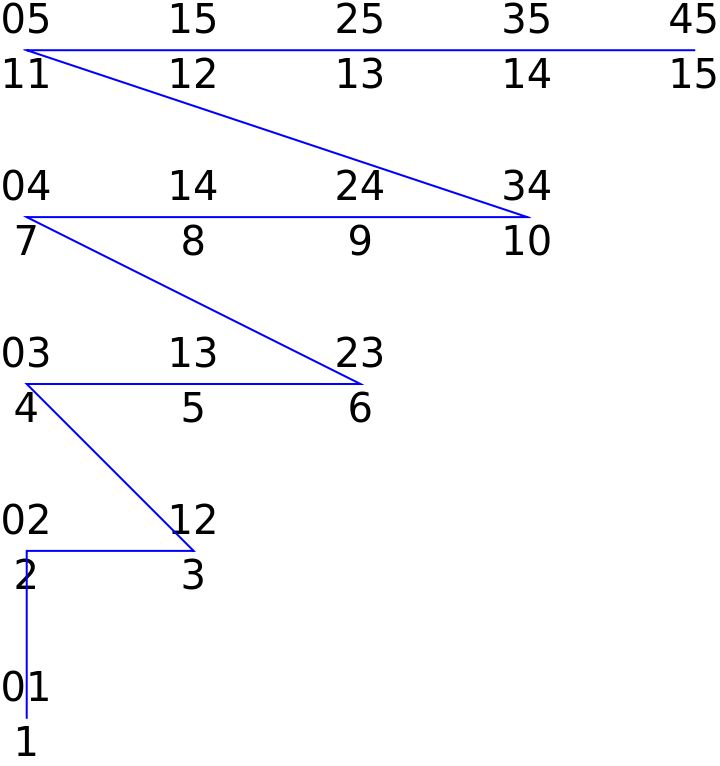The structure of 3-subsets in 3D:

 In:=Out=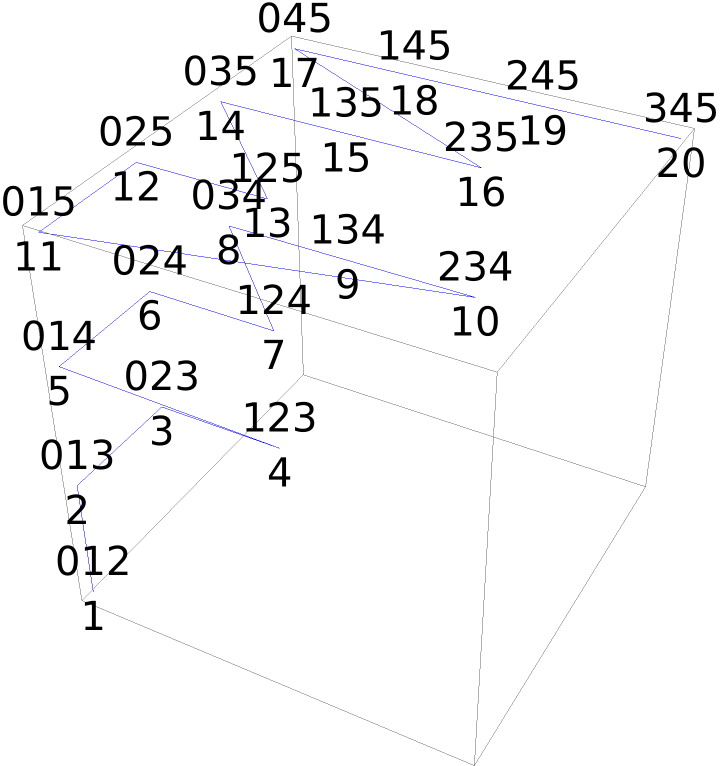### Neat Examples (1)

Find the trillionth numbers with binary weights eight to twelve:

 In:=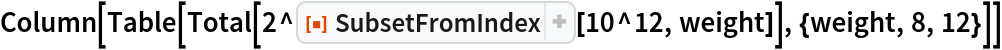Out=## Version History

• 2.0.0 – 24 December 2019
• 1.0.0 – 05 December 2019